# C++ Programming Examples: Basic Input & Output stream objects, cin and cout

(Last Updated On: April 4, 2021)

## cin C++ Stream object Programming Examples:

Output:

Output:

Output:

### C++ Programming Example 4: Example write a program that inputs the name, age, height and gender of a student using ‘cin C++ stream object’:

#### Flow chart: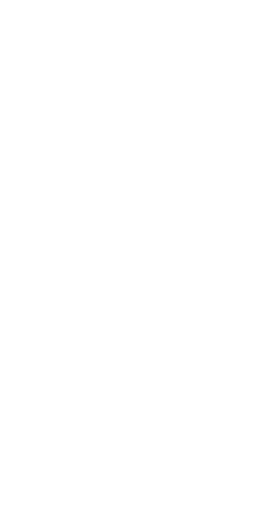#### Program: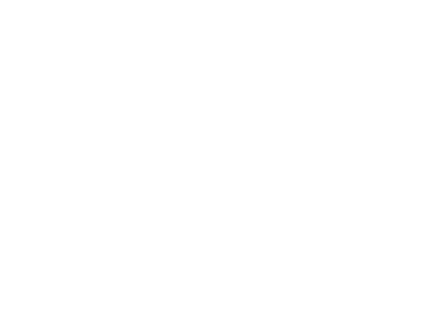### C++ Programming Example 5: write a program that inputs distance in miles from user and converts miles into kilometers. One mile =1.609 kilometer using cin cout stream objects: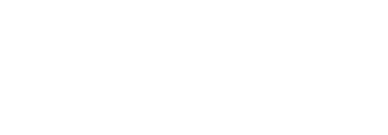### C++ Programming Example 6: write a program in C++ to converts pound into kilograms using cin cout stream objects:

1 pound = 0.453592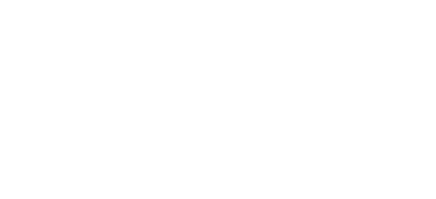### C++ Programming Example 7: write a program in C++ which convert Inches to centimeters using cin cout stream objects:

1 inch = 2.54 centimeters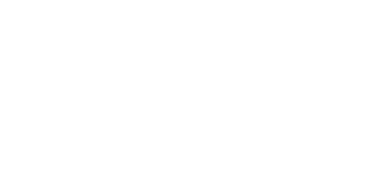### C++ Programming Example 8: writes a program in C++ to calculate the volume and surface area of a sphere using cin cout stream objects:

Formula:

area of sphere = 4π R2
circumference f sphere = 4/3 πR3
the value of π is = 3.1417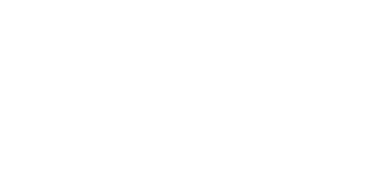### C++ Programming Example 9: write a program in C++ which calculate the area and circumference of the circle using cin cout stream objects:

Formula:

area of circle = π R2
circumference of circle= 2πR
the value of π is = 3.1417

#### Flowchart: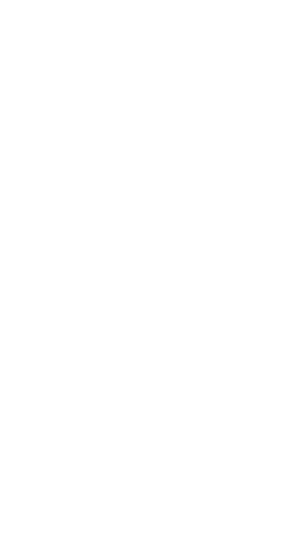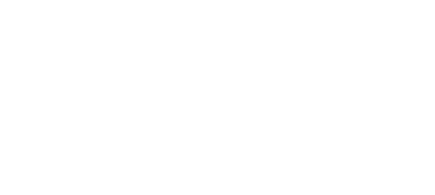### C++ Programming Example 10: write a temperature program in C++ which converts the Fahrenheit to Centigrade using cin cout stream objects:

C=5/9(F-32)

#### Flowchart: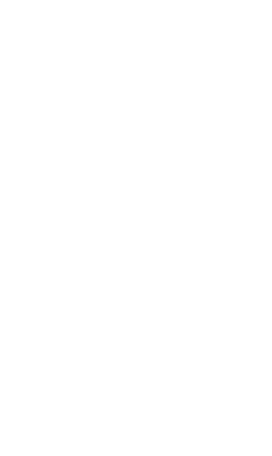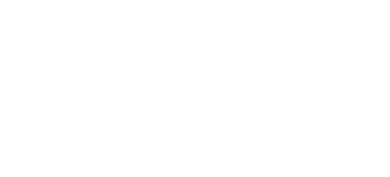### C++ Programming Example 11: write a program that inputs marks obtained by a student in five different subject and find out aggregate marks (total marks) and percentageusing cin cout stream objects: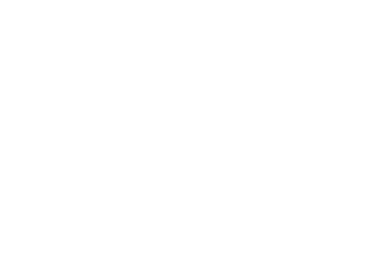### C++ Programming Example 12: write a program that inputs the value of three sides of a triangle and finds out its areausing cin cout stream objects:

Formula: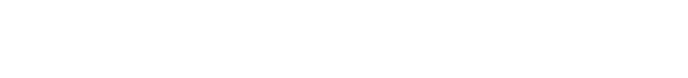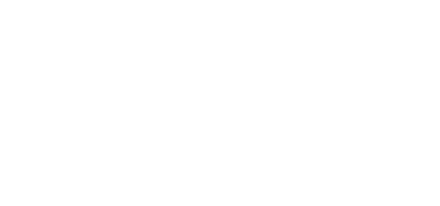### C++ Programming Example 13: write a program which consists of three variable Area, Perpendicular, and Base. The program inputs the values in variables per and base from the user and computes the area of a triangleusing cin cout stream objects:

Formula:

Area = (base*per)/2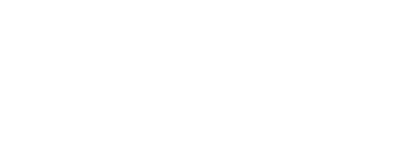### C++ Programming Example 14: write a program in C++ which reverse the order of the long numbersusing cin cout stream objects: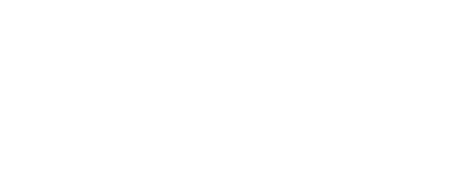### C++ Programming Example 15: Write a program that inputs the current price of gas and electricity per unit. Give each item’s rate with an increment of 20%. The program computes the new price of gas and electricity using cin cout stream objects: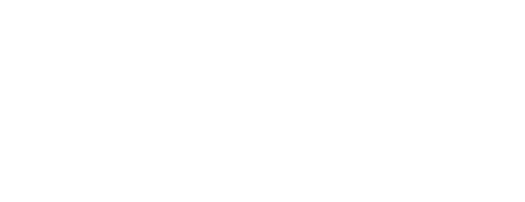### C++ Programming Example 16: write a program which calculate the employee dearness allowance, medical allowance, house rent allowance and calculate the gross salary and displayusing cin cout stream objects:

25% dearness allowance.

15% medical allowance.

45% house rent allowance.

formula:

gross salary= basic pay + dearness allowance + medical allowance + house rent.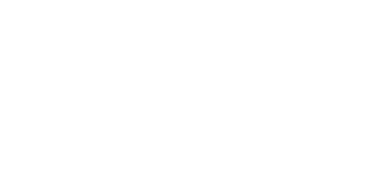## The ‘cout’ C++ Stream Object:

The cout is pronounced as See Out. The cout stands for console output. The cout is a c++ standard output C++ stream object. It is used as an output statement to display.

Output on the computer screen. This C++ stream object represents the flow of data from computer memory to display screen. It is a part of iostream.h header file.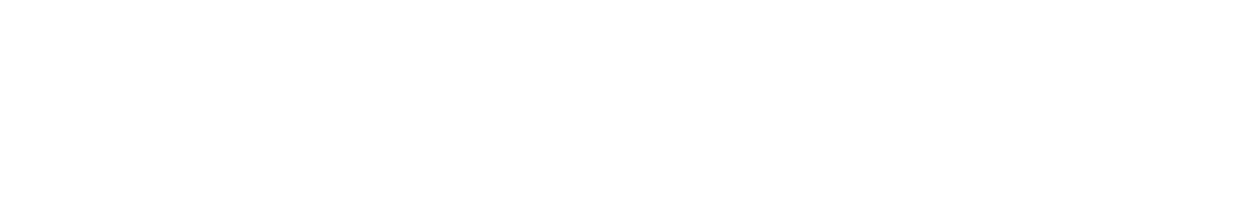The general syntax of cout is as follows:

cout<<const1/var1/exp1[<<consta2/var2/exp2/….];

where

cout:

it is the name of output C++ stream object, which is used to display the standard output.

<<:

It is known as insertion operator or put to operator. It sends the output (contents of variable or constant) to the cout object. The cout object then sends the output to the display screen. We can use multiple insertion operators to display the valued of multiple variables or constants.

Const1/var1/exp1, Const2/var2/exp2:

It indicates the list of constants, variables or arithmetic. Expression. for each constants, or variable or expression. separated insertion operator (<<) is used. The string constant is given in double quotation marks. Character constant is given in singe quotation marks. However, variables, numeric constants and expression are given without quotation marks.

Examples:

• Following statement will display a message “Programming Digest”:

Cout<<”Programming Digest”;

This output statement consists of only one string constant which is to be displayed on the screen. This string constant is given in double quotation marks.

• Following statement will display the value of variable ‘sum’ on the screen.

cout<<sum;

Please note that in this output statement the variable ‘sum’ is not enclosed with any quotation marks.

• Following statement uses one variable “km”, two string constants, one arithmetic expression and four insertion operators:

cout<<km<<” kilometers= “<< km*1000<<”meter”;

Suppose the value of km is 6. The output of the above output statement will be:

3 kilometers= 3000

### C++ Programming Example 17: write a program that computes and display the area of a square. The formula to compute the area of square isusing cout stream object:

Area = height * width;

#### Flowchart: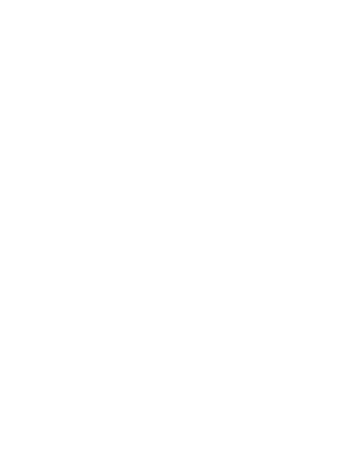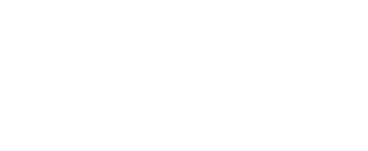It the above program “Area of square =” is a text message. The text message will be displayed first and the value of variable ‘area’ will be displayed.

### C++ Programming Example18: write a program that adds two floating point values and displays the sum on the screenusing cout stream object: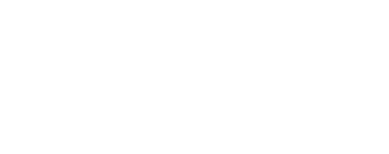### C++ Programming Example19: write program that converts 45.6 centigrade temperature into Fahrenheit using the formula F=9/5(C+32) and displays the temperature in Fahrenheitusing cout stream object: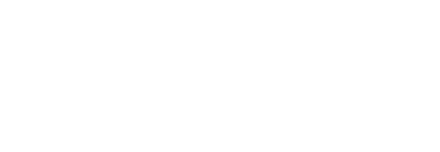### C++ Programming Example20: write a program which convert age in moths and daysusing cout stream object: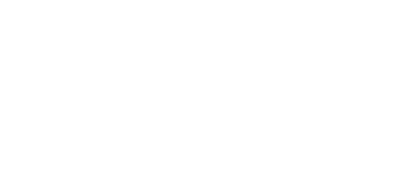### C++ Programming Example21: write a program that displays values of integer, floating point variable and character variable on the screenusing cout stream object: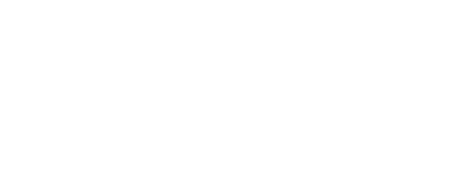### C++ Programming Example22: write a program that calculates the distance covered by a body in 20.5 seconds. The initial velocity of body is 15.8 meter per second and acceleration is 10.2m/sec2using cout stream object:

Formula:

S=vit+1/2at2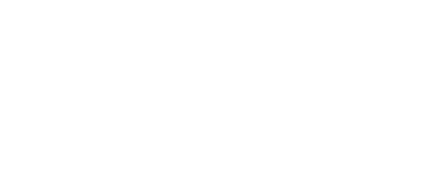Top Gaming Computers

Best Laptops

Best Graphic Cards

Portable Hard Drives

Best Keyboards

Best High Quality PC Mic

Computer Accessories